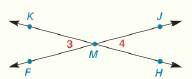Chapter 1.CR, Problem 26CR### Elementary Geometry for College St...

6th Edition
Daniel C. Alexander + 1 other
ISBN: 9781285195698

#### Solutions

Chapter
Section### Elementary Geometry for College St...

6th Edition
Daniel C. Alexander + 1 other
ISBN: 9781285195698
Textbook Problem
1 views

# Given: m ∠ F M H = 4 x + 1 m ∠ 4 = x + 4 Find: m ∠ 4To determine

To find:

The value of m4.

Explanation

Given:

mFMH=4x+1

And,

m4=x+4

The Figure (1) is given as,

Figure (1)

Definition:

The pair of nonadjacent angles in the opposite direction that lie on two intersecting straight lines is called vertical angles.

Calculation:

The angle m4 can be written as,

mFMH+m4=180°(1)

Substitute x+4 for m4 and 4x+1 for mFMH in equation (1).

### Still sussing out bartleby?

Check out a sample textbook solution.

See a sample solution

#### The Solution to Your Study Problems

Bartleby provides explanations to thousands of textbook problems written by our experts, many with advanced degrees!

Get Started

#### Find more solutions based on key concepts